• 0

# Central Limit Theorem, Law of Large Numbers

Go to solution Solved by Atul Dev,

Central Limit Theorem

Central Limit Theorem (CLT) - states that for multiple samples taken from a population (with known mean and variance), if the sample size is large, then the distribution of the sample mean, or sum, will converge to a normal distribution even though the random variable x (individual data points within a sample) may be non-normal. This proves to be a key concept in probability theory as it implies that probabilistic and statistical methods that work for normal distributions can be applicable to many problems involving other types of distributions. It usually gives the below conditions

1. Sample means always follow normal distribution irrespective of distribution of individual data in population
2. Mean of sample means tends to population mean as the number of samples tend to infinity
3. Variance of sample means is 'n' times less than the variance of population, where 'n' is size of sample
e.g. Consider the roll of 2 dice. If this is done multiple times and the average or the sum of the rolls is plotted, then this plot will converge to a normal distribution

Law of Large Numbers - states that as sample size grows, the sample mean gets closer to the population mean irrespective whether the data set is normal or non-normal e.g. consider the roll of a single dice. If you roll the dice sufficiently large number of times, the average would tend to be close to 3.5.

An application oriented question on the topic along with responses can be seen below. The best answer was provided by Atul Dev on 15th September 2017.

## Question

Q9. What is the difference between Central Limit Theorem and Law of Large Numbers? If central limit theorem can help us achieve a normal distribution, why should we not always make use of it and get rid of Non-Normal data?

Note for website visitors - Two questions are asked every week on this platform. One on Tuesday and the other on Friday.

## Recommended Posts

• 1
• Solution

ReaCentral Limit Theorem (CLT) gives us three conditions:

1) Mean of sample means tends to population mean as the number of samples tend to infinity.

2) Sample means always follow normal distribution irrespective of distribution of individual data in population.

3) Variance of sample means is 'n' times less than the variance of population, where 'n' is size of sample.

Law of Large Numbers (LLN) states that as the sample size grows, its mean gets closer to the average of the whole population.

Here it can be noted that CLT talks about 'Mean of Sample Means approaching Population Mean' whereas LLN talks about 'Mean of Large Sample approaching Population Mean'. So there is a difference in both the approaches. Practically, in Statistical Quality Control (SQC), it is sometimes convenient to deal with grouped samples, and for this purpose, CLT provides us a powerful tool to draw inferences about the population.

CLT makes 'non-normal' data 'normal' only if we are dealing with sample averages. In case we have to deal with the population data directly, which is not normally distributed, then CLT will not help us.

##### Share on other sites
• 1

Central Limit theorem states that irrespective of whether the population follows normal distribution or not, sample averages pulled from the population will always follow Normal distribution.

The Law of Large numbers states that the frequencies of events with the same likelihood of occurrence even out when we see over a large number of trials. i.e. as sample grows larger the outcomes (of interest) will tend towards the Expected value.

We can make use of the CLT when ever we have a situation where a sample mean which spreads over the frequencies of occurrences is available and relevant. Several statistical methods and tests do make use of this principle. Popular one is the control chart.

However there are non-normal situations where it may not be practical or relevant to have such sample means.

For example failure data are represented by Exponential distribution, which is non-normal. Here the frequencies are distributed over time period. One cannot expect to take sample values representing the time spread and use them for reliability prediction and analysis for improvements.

There are many situations where the presence of non-normality in the population is an indication of certain abnormality that needs to be identified and addressed. For example a multi peaked distribution of a quality characteristic on a lot received from a vendor could indicate mix up of the lots from two populations. A skewed  distribution may represent a screened population. Such information should not get camouflaged by picking up sample means and associating with treatments meant for normal distribution.

To conclude, while the CLT is a powerful concept that has its sphere of application, the studies and treatments for Non-Normal distributions have their importance as well depending on the context.

##### Share on other sites
• 0

Law of Large Numbers states that: sample average converges to the expected average as the sample size goes to infinity.

Central Limit Theorem states that: as sample size goes to infinity, the sample mean distribution will converge to a normal distribution.

Having to deal with Non-normal data is quite a normal and a common phenomenon, the reasons why we face non-normal data is because there will be measurement errors, data-entry errors, outliers, there can be overlap of two or more processes and incorrectly assuming normality can be risky.

There are many data types that follow a non-normal distribution by nature. Examples include:

·         Weibull distribution, found with life data such as survival times of a product

·         Log-normal distribution, found with length data such as heights

·         Largest-extreme-value distribution, found with data such as the longest down-time each day

·         Exponential distribution, found with growth data such as bacterial growth

·         Poisson distribution, found with rare events such as number of accidents

·         Binomial distribution, found with “proportion” data such as percent defectives

If data follows one of these different distributions, it must be dealt with using the same tools as with data that cannot be “made” normal.

References:

1)      Isixsigma

2)      Bugra.github

##### Share on other sites
• 0

CENTRAL LIMIT THEOREM  refines the LAW OF LARGE NUMBERS

The Law of large numbers gives the conditions under which sample moments converge to population moments as sample size increases

While The cenrral limit theorem provides information about the rate at which sample moments converge to population moments as sample size increases

Regarding second part of question

William Watt said, “Do not put your faith in what statistics say until you have carefully considered what they do not say.

Some of the examples which might create HAVOC while applying central limit theorem are

1) If you have a perfect distribution of data on monday 2:00 PM, it has a little bearing on being representative of wednesday afternoon

2) we sometimes stuck with the data which is changing or shifting with respect to internal or any external factors with time. In this case data from the earlier period becomes less representative of current conditions.

3) It also depend on data is whether Biased or Unbiased. As with the Biased data we will have different proportions of actions from each unique group. If we biased our data with some invalid reasoning/ fault move which can leads to the conclusion that is not much relevant to the real world..

4) There are always going to be some mechanical issues which add noise factor in population. No matter which data acquisition technology we are using. As much i am aware we dont have perfect data capturing methods as we use representations of representations

HAVE A NICE DAY!

REGARDS

ATUL SHARMA

##### Share on other sites
• 0

The Central limit Theorem states that when sample size tends to infinity, the sample mean will be normally distributed.

The Law of Large Number states that when sample size tends to infinity, the sample mean equals to population mean.

The two statements are not contradictory.

The Central Limit Theorem tell us that as the sample size tends to infinity, the of the distribution of sample means approaches the normal distribution. This is a statement about the SHAPE of the distribution. A normal distribution is bell shaped so the shape of the distribution of sample means begins to look bell shaped as the sample size increases.

The Law of Large Numbers tells us where the center (maximum point) of the bell is located. Again, as the sample size approaches infinity the center of the distribution of the sample means becomes very close to the population mean.

When data is not normally distributed, the cause for non-normality should be determined and appropriate remedial actions should be taken. There are six reasons that are frequently to blame for non-normality.

#### Reason 1: Extreme Values

Too many extreme values in a data set will result in a skewed distribution. Normality of data can be achieved by cleaning the data. This involves determining measurement errors, data-entry errors and outliers, and removing them from the data for valid reasons.

It is important that outliers are identified as truly special causes before they are eliminated. Never forget: The nature of normally distributed data is that a small percentage of extreme values can be expected; not every outlier is caused by a special reason. Extreme values should only be explained and removed from the data if there are more of them than expected under normal conditions.

#### Reason 2: Overlap of Two or More Processes

Data may not be normally distributed because it actually comes from more than one process, operator or shift, or from a process that frequently shifts. If two or more data sets that would be normally distributed on their own are overlapped, data may look bimodal or multimodal – it will have two or more most-frequent values.

The remedial action for these situations is to determine which X’s cause bimodal or multimodal distribution and then stratify the data. The data should be checked again for normality and afterward the stratified processes can be worked with separately.

An example: The histogram in Figure 2 shows a website’s non-normally distributed load times. After stratifying the load times by weekend versus working day data (Figure 3), both groups are normally distributed.

Figure 2: Website Load Time Data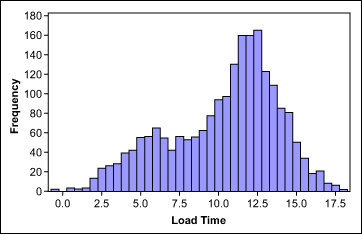Figure 3: Website Load Time Data After Stratification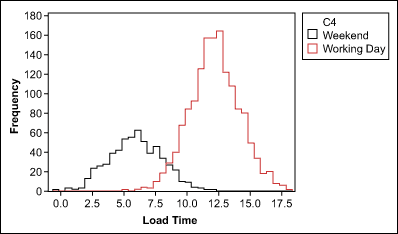#### Reason 3: Insufficient Data Discrimination

Round-off errors or measurement devices with poor resolution can make truly continuous and normally distributed data look discrete and not normal. Insufficient data discrimination – and therefore an insufficient number of different values – can be overcome by using more accurate measurement systems or by collecting more data.

#### Reason 4: Sorted Data

Collected data might not be normally distributed if it represents simply a subset of the total output a process produced. This can happen if data is collected and analyzed after sorting. The data in Figure 4 resulted from a process where the target was to produce bottles with a volume of 100 ml. The lower and upper specifications were 97.5 ml and 102.5 ml. Because all bottles outside of the specifications were already removed from the process, the data is not normally distributed – even if the original data would have been.

Figure 4: Sorted Bottle Volume Data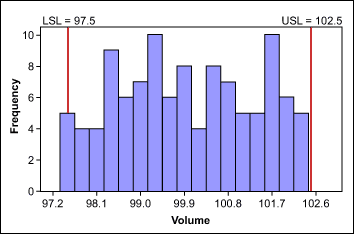#### Reason 5: Values Close to Zero or a Natural Limit

If a process has many values close to zero or a natural limit, the data distribution will skew to the right or left. In this case, a transformation, such as the Box-Cox power transformation, may help make data normal. In this method, all data is raised, or transformed, to a certain exponent, indicated by a Lambda value. When comparing transformed data, everything under comparison must be transformed in the same way.

The figures below illustrate an example of this concept. Figure 5 shows a set of cycle-time data; Figure 6 shows the same data transformed with the natural logarithm.

Figure 5: Cycle Time Data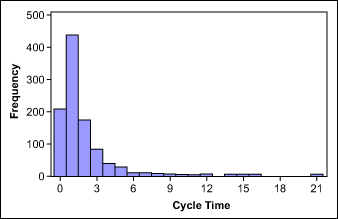Figure 6: Log Cycle Time Data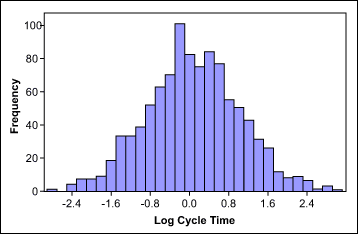Take note: None of the transformation methods provide a guarantee of a normal distribution. Always check with a probability plot to determine whether normal distribution can be assumed after transformation.

#### Reason 6: Data Follows a Different Distribution

There are many data types that follow a non-normal distribution by nature. Examples include:

• Weibull distribution, found with life data such as survival times of a product
• Log-normal distribution, found with length data such as heights
• Largest-extreme-value distribution, found with data such as the longest down-time each day
• Exponential distribution, found with growth data such as bacterial growth
• Poisson distribution, found with rare events such as number of accidents
• Binomial distribution, found with “proportion” data such as percent defectives

If data follows one of these different distributions, it must be dealt with using the same tools as with data that cannot be “made” normal.

### No Normality Required

Some statistical tools do not require normally distributed data. To help practitioners understand when and how these tools can be used, the table below shows a comparison of tools that do not require normal distribution with their normal-distribution equivalents.

 Comparison of Statistical Analysis Tools for Normally and Non-Normally Distributed Data Tools for Normally Distributed Data Equivalent Tools for Non-Normally Distributed Data Distribution Required T-test Mann-Whitney test; Mood’s median test; Kruskal-Wallis test Any ANOVA Mood’s median test; Kruskal-Wallis test Any Paired t-test One-sample sign test Any F-test; Bartlett’s test Levene’s test Any Individuals control chart Run Chart Any Cp/Cpk analysis Cp/Cpk analysis Weibull; log-normal; largest extreme value; Poisson; exponential; binomial
##### Share on other sites
• 0

Central Limit Theorem states that if a sufficiently large sample size is drawn from the population with limited level of variance, mean of all samples from same population will be approximately equal to the mean of the population. Also repeatedly taking independent random samples of size n from population, then when n is large, distribution of the sample mean will approach normal distribution.

Central Limit Theorem talks about the distribution of the sample data in a large population. Where as law of large numbers talk about law of averages relating to likelihood of occurrence of the events in case of high frequency of events.

Practically its not feasible to go for large population of the data due to associated cost, so its not practically possible to make use of central limit theorem for getting rid of non-normal data.

##### Share on other sites
• 0

 S.No Central limit theorem Law of large numbers 1. Sample mean will be normally distributed Sample mean equals to population mean 2. Can be executed for less data Can be executed for very large numbers 3. It will not converge to a number, it converges to a normal distribution. Sample mean will converge to a Number 4. Gives value and distribution of sample mean for n numbers Gives value and distribution of sample mean for n numbers 5. The standard deviation of the sample means equals the standard deviation of the population Standard deviation declines as the size of population or sample increases

When data is not normally distributed, the cause for non-normality should be determined and appropriate remedial actions should be taken, without that we cannot change every non normal data to normal data by using central limit theorem.

1.        Too many extreme values in a data set will result in a skewed distribution. Normality of data can be achieved by cleaning the data. This involves determining measurement errors, data-entry errors and outliers, and removing them from the data for valid reasons.

2.        Round-off errors or measurement devices with poor resolution can make truly continuous and normally distributed data look discrete and not normal.

Data errors should be taken care to change the non normal data to normal data

##### Share on other sites
• 0

Two very important theorems in statistics are the Law of Large Numbers and the Central Limit Theorem.

1. The Central limit Theorem (CLT) states that when sample size tends to infinity, the sample mean will be normally distributed.
2. The Law of Large Number (LLN) states that when sample size tends to infinity, the sample mean equals to population mean.

CLT establishes the normal distribution as the distribution to which the mean (average) of almost any set of independent and randomly generated variables rapidly converges. It also give precise values for the mean and standard deviation of the normal variable. It is generally an excellent approximation for the mean of a collection of data (often with as few as 10 variables).

LLN establishes that as the number of identically distributed, randomly generated variables increases, their sample mean (average) approaches their theoretical mean. For example, if the measuring device is defective or poorly calibrated then the average of many measurements will be a highly accurate estimate of the wrong thing.

The CLT says that as the sample size tends to infinity, the distribution of mean approaches the normal distribution. This is a statement about the SHAPE of the distribution. A normal distribution is bell-shaped so the shape of the distribution of sample means begins to look bell-shaped as the sample size increases.

The LLN tells us where the centre (maximum point) of the bell is located. Again, as the sample size approaches infinity the centre of the distribution of the sample means becomes very close to the population mean.

CLT requires extra assumptions on top of those needed for LLN. So you can have LLN without CLT but not the other way around.

##### Share on other sites
• 0

The Central limit Theorem states that when sample size tends to infinity, the sample mean will be normally distributed.

The Law of Large Number states that when sample size tends to infinity, the sample mean equals to population mean.

The LLN gives conditions under which sample moments converge to population moments as sample size increases

The CLT provides information about the rate at which sample moments converge to population moments as sample size increases

The law of large numbers says that the mean of a sample of a random variable's values equals the true mean μ as N goes to infinity, then it seems even stronger to say that (as the central limit says) that the value becomes N(μ,σ)N(μ,σ) where σ is the standard deviation.

##### Share on other sites
• 0

Central Limit Theorem (CLT) states that, as the sample size tends to infinity the distribution of sample means approaches the normal distribution i.e. a bell shaped curve. So, in other words, this theorem talks about the shape of the distribution of sample mean, as sample size tends to infinity.

The Law of Large Numbers states that, as the sample size tend to infinity, the centre of the distribution (mean) of the sample-means becomes very close to the population mean. So, in other words, this theorem talks about where the centre (maximum point) of the bell curve is located.

Example: Consider a large population and you need to measure the average height of men in the whole population. i.e. we need to calculate the population mean(µ).

It may not be humanly possible to measure height of each men to compute the average. So, we can consider creating "N" sample sets (s1, s2, s3…sN) of “n” men each. i.e. sample size is “n”.

These sample sets should be independent and identically-distributed which means it should be random and occurrence should be independent. A sample should not be taken , say, of just Basketball players, whose height would be above that of average men. If Basket players are considered, then they should occur in every sample set. (just argument sake). Usually, if we know that people were selected randomly, then we can assume that the independence assumption is met.

Sample 1: s1(height of “n” men) Sample-Mean (i.e.mean of Sample set 1) : x1

Sample 2: s2(height of “n” men) Sample-Mean: x2

Sample 3: s3(height of “n” men) Sample-Mean: x3

.

Sample N: sN(height of “n” men) Sample-Mean: xN

[Total number of such samples “N” required depends on the population size and is a separate topic of research.]

Per Central Limit Theorem, as “n” --> ∞ (infinity), the plot of Sample-means x1, x2, x3…xN forms a normal distribution or Bell curve.

Per Law of Large Numbers, as “n” --> ∞ (infinity), the centre (maximum point) /mean of this bell curve would be close to the population mean (µ) that we need to calculate. i.e. (x1+x2+x3+....+XN)/N is close to µ

As sample size “n” is increased (“n” --> ∞), the bell curve becomes narrower i.e. the standard deviation between sample means reduces and sample-means get closer to the population mean.

Why not get rid of Non-Normal data?

Most of the sample data or data sets available for analysis may not be normally distributed.

1. Data obtained from overlap/combination of two process may not be normally distributed even if, the individual process data may be normally distributed.
2. Round-off errors or measurement devices with poor resolution can make normally distributed data look discrete and not normal.
3. Collected data might not be normally distributed if it is a subset of the total output data produced from a process. This can happen if data is collected and analysed after sorting.

So more than often we must deal with Non-Normal data. Using the CLT to get a normal distribution uses the following assumptions and conditions:

1. Random Samples: The data must be sampled randomly.
2. Independent and Identically-distributed samples: The sample values must be independent of each other. This means that the occurrence of one event has no influence on the next event.
3. 10% Condition: When the sample is drawn without replacement (usually the case), the sample size, n, should be no more than 10% of the population.
4. Sample Size Assumption: The sample size must be sufficiently large. The Central Limit Theorem states that a Normal distribution model can be used to think about the behavior of sample means when the sample size is large enough, but it does not specify how large it should be. If the population is very skewed, then a pretty large sample size will be needed to use the CLT. However, if the population is unimodal and symmetric, even small samples would work. The sample size should be decided (i.e. whether large enough) in terms of information that can be obtained regarding the population. In general, a sample size of 30 is considered sufficient if the sample is unimodal (and meets the 10% condition).

These assumptions and conditions make it difficult to apply CLT under all circumstances. Depending on the population size and sample size the approximations may give inaccurate results. So it is not a possible option  to get rid on Non Normal data completely.

##### Share on other sites
• 0

Central Limit Theorem

The Central Limit Theorem, states that any data set that is randomly sampled from a population, regardless of distribution, will be approximately normal IF the sample size is large.

This means that we can treat any data as normal, as long as we sample enough times from the population.

But How Large is Large?

Well, we say large enough, but what do we really mean by that?

For most cases, any sample size of at least 30 will allow the CLT to be applied. Certain special cases exist, but this is a good rule to follow.

Example:

A business client of FedEx wants to deliver urgently a large freight from Denver to Salt Lake City. When asked about the weight of the cargo they could not supply the exact weight, however they have specified that there are total of 36 boxes.

You are working as a Business analyst for FedEx. And you have been challenged to tell the executives quickly whether or not they can do certain delivery.

Since, we have worked with them for so many years and have seen so many freights from them we can confidently say that the type of cargo they follow is a distribution with a mean of μ= 72 lb (32.66 kg) and a standard deviation of σ = 3 lb (1.36 kg).

The plane you have can carry the max cargo weight upto 2640 lb (1193 kg). Based on this information what is the probability that all of the cargo can be safely loaded onto the planes and transported?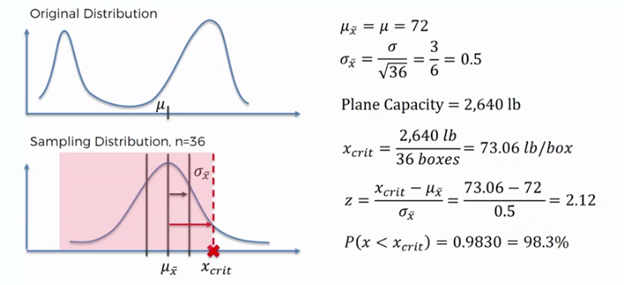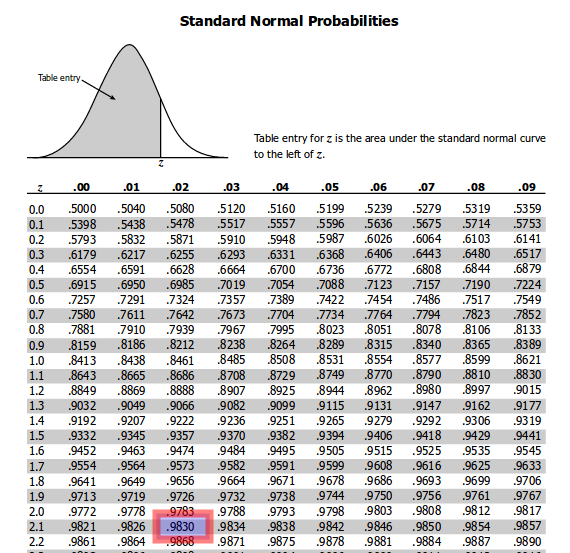Now, you can go to the manager and tell him that I have done the calculations and the probability that the plan can safely takeoff is 98.3% and 1.7 % chance it cannot takeoff.

Law of Large Numbers:

Have you ever seen a contest where there is a jar full of jelly beans along with a prize for the person who guesses how many jelly beans there are inside?

If you try to guess, your answer may not come too close to the total number of jelly beans in the jar. The same may be true if you average the guesses of ten people who give it a try, but what happens if 1,000 people each take a guess and we average their guesses? Interestingly, that average will likely be a lot closer to the actual number of jelly beans in the jar.

Taking it further, if 10,000 people take a guess and we average their guesses, that number will get even closer to the actual number of jelly beans in the jar. Which means the probability of guessing the correct amount of jelly beans is higher. As a matter of fact, as the number of guesses increases, the average of the guesses will come closer and closer to the actual number of jelly beans. This is the law of large numbers in action!

Why are we not making use of CLT?

Application of Central Limit Theorem is little tricky, why? Because of the size of the samples would keep on increases with the higher standard deviation and with the type of distribution. In such instances – it’s practically difficult for us to collect more data points as it consumes more time, eventually changes to the process would also be more.

references:

study.com

medium.com

##### Share on other sites
• 0

Central Limit Theorem - The means of randomly selected independent samples from a population distributes themselves normally. This holds true even when the population doesn’t align as a bell curve. Another element of the theory suggests that as the size of the samples increases, the distribution of the means becomes less spread out.

Skewed populations require larger samples when compared to normally distributed ones. A thumb rule of 30 samples should make one comfortable with the distribution.

Law of Large numbers - A rule where in when the experiment is carried out enough times one does end up with the average/expected probability. The coin when tossed, the recorded outcome post many trails will lead to the expected probability of 50% each side.

Both complement each other. The Central Limit theorem closes in on Law of large numbers.  CLT advises about the rate while LLN provides the parameters of the sample means that converge to population means when the sampling increases.

Normal and Non Normal Data – Spread of the data points in an investigating can shape into a symmetrical inverted bell or skewed to either side of the graph. The former is considered to be normal and the latter is non normal.

For normal data, parametric tests are used and they have higher power (used in hypothesis testing for inference) when compared to the non parametric tests carried out on the non normal distribution of similar sample size. Non parametric test are more robust (insensitivity to the violations of assumptions) and the corresponding conclusions are more accurate when compared to the same test through parametric tests.

Both the tests hold good depending on the sample size availability, the power needed to infer about the population and the risk with assumptions and groups.

Before “transforming” skewed to normal and then using parameter testing – one has to study the data and the underlying message of the sample. For instance, if the sample has outliers, its quite naive to resort to truncation/transformation rather than analyzing the special cause of the extreme data point. When the sample is inherently non normal, one has to administer caution by considering sample size and trade offs with power and flexibility.

##### Share on other sites
• 0

Central Limit Theorem:

It states that when sample size tends to infinity, the sample mean will be normally distributed.

Expanding this with a standard wiki definition

“Central limit theorem, establishes that, in most situations, when independent random variables are added, their properly normalized sum tends toward a normal distribution (a bell curve) even if the original variables themselves are not normally distributed”

Law of Large Numbers:

It states that when sample size tends to infinity, the sample mean equals to population mean.

Put in other words, when the quantity/size of the identically distributed, randomly generated variables increases, their sample mean (average) equals the population mean

Difference Between Central Limit Theorem and Law of Large numbers :

 Central Limit Theorem Law of Large Numbers When sample size increases then the sample mean will be normally distributed When sample size increases, the sample mean equals the population mean. It normally takes a size of over 30, for the distribution to become a normal distribution There is no specification for minimal size for this to be realised The theorem talks about the shape of the distribution The theorem talks about the centre point of the bell shaped curve

Why should we not always make use of Central Limit Theorem (CLT) and get rid of Non-Normal data?

1. There may be a scenarios where getting a considerable sample size (good enough to do) may not be prevalent for doing normal distribution

2. Also one will not know whether the sample size is “large enough”  to have normalisation. How large is that? In order to understand that the required sample size varies for different sorts   of data,  simulations are the way to go which will reflect the kind of data that is being used

##### Share on other sites
• 0

While Central limit theorem or CLT can help us achieve a normal distribution, however, to achieve the same one can encounter a few issues which would need to be addressed:

1. Extreme values in data or outliers
2. Data following a different distribution curve
3. Values are close to 0

Hence, in case the data is non-normal due to the right reasons there are other statistical tools which can be used in those cases.

##### Share on other sites
• 0

Central Limit Theorem and Law of Large Numbers

The Central Limit Theorem tells us that as the sample size tends to infinity, the distribution of sample means approaches the normal distribution. This is a statement about the shape of the distribution. A normal distribution is bell shaped so the shape of the distribution of sample means begins to look bell shaped as the sample size increases. In other words, as sample size goes to infinity, the sample mean distribution will converge to a normal distribution.

The Law of Large Numbers tells us where the centre (maximum point) of the bell is located. The sample size approaches infinity the centre of the distribution of the sample means becomes very close to the population mean. In other words, the average of independent (many) samples will converge to the mean of the underlying distribution that the observations are sampled from.

The Central Limit Theorem describes the relation of a sample mean to the population mean.  If the population mean doesn't exist, then the CLT doesn't apply and the characteristics of the sample mean, Xbar, are not predictable. We can always compute the numerical mean of a  finite number of observations from any density (if every observation is finite).  But the population mean is defined as an integral, which diverges, so even though a sample mean is finite, the population mean is not. The distribution of the sample average is that same as the distribution of an individual observation, so the scatter never diminishes, regardless of sample size.  The Central Limit Theorem almost always holds, but it’s application needs caution. If the population mean doesn't exist, then the CLT is not applicable.  Further, even if the mean does exist, the CLT convergence to a normal density might be slow, requiring hundreds or even thousands of observations, rather than the few dozen in these examples. Therefore, we cannot get rid of non-normal data set always.

##### Share on other sites
• 0

The Central limit theorem tells that as the sample size tends to infinity,the distribution of sample means approaches the normal distribution.So it's about the shape of distribution as normal distribution is bell shaped .So the shape of distribution of sample means begins to look bell shaped as the sample size increases.

The Law of Large numbers tells where the centre of the bell is located.As the sample size approaches infinity the centre of the distribution of the sample means becomes very close to the population mean.

The CLT requires the data to be independent and identically distributed.It holds good under the suitable assumption of short tails or finite moments.Under serial dependence CLT deteriorates and fails for certain long memory processes.

##### Share on other sites
• 0

The Central Limit Theorem tell us that as the sample size tends to infinity, the of the distribution of sample means approaches the normal distribution. This is a statement about the SHAPE of the distribution. A normal distribution is bell shaped so the shape of the distribution of sample means begins to look bell shaped as the sample size increases.

The Law of Large Numbers tells us where the centre (maximum point) of the bell is located. Again, as the sample size approaches infinity the centre of the distribution of the sample means becomes very close to the population mean.

I will take this to mean that it is the belief that for i.i.d. random variables Xi with mean μ and standard deviation σ, the cumulative distribution function FZn(a) of

Zn=1n∑i=1nXi

converges to the cumulative distribution function of N(μ,σ), a normal random variable with mean μ and standard deviation σ. Or, the minor re-arrangements of this formula, e.g. the distribution of Zn−μ converges to the distribution of N(0,σ), or the distribution of (Zn−μ)/σ converges to the distribution of N(0,1), the standard normal random variable. Note as an example that these statements imply that

P{|Zn−μ|>σ}=1−FZn(μ+σ)+FZn((μ+σ)−)→1−Φ(1)+Φ(−1)≈0.32

as n→∞.

The weak law of large numbers says that for i.i.d. random variables Xi with finite mean μ, given any ϵ>0,

P{|Zn−μ|>ϵ}→0  as n→∞.

Note that it is not necessary to assume that the standard deviation is finite.

The central limit theorem does not imply the weak law of large numbers. As n→∞, the other version of the central limit theorem says that P{|Zn−μ|>σ}→0.317 while the weak law says that P{|Zn−μ|>σ}0

From a correct statement of the central limit theorem, one can at best deduce only a restricted form of the weak law of large numbers applying to random variables with finite mean and standard deviation. But the weak law of large numbers also holds for random variables such as Pareto random variables with finite means but infinite standard deviation.

I think it is safe to say that the sample mean converges to a normal random variable with nonzero standard deviation is a stronger statement than saying that the sample mean converges to the population mean, which is a constant (or a random variable with zero standard deviation if you like). Isn’t it?

##### Share on other sites
• 0

The Central Limit Theorem tell us that as the sample size tends to infinity, the distribution of sample means approaches the normal distribution. This is a statement about the SHAPE of the distribution. A normal distribution is bell shaped so the shape of the distribution of sample means begins to look bell-shaped as the sample size increases. Law of Large Numbers says if we have very large sample, the mean will converge to a number.

The Law of Large Numbers tells us where the center (maximum point) of the bell is located. Again, as the sample size approaches infinity the center of the distribution of the sample means becomes very close to the population mean. Central Limit Theorem requires "less data" Comparing to Law of Large Numbers, because it require "less data", it has a relaxation in conclusion: not converge to a number, it converge to a normal distribution.

In the application of central limit theorem to sampling statistics, the key assumptions are that the samples are independent and identically distributed.

##### Share on other sites
• 0

The Central limit theorem states that when sample size tends to infinity, the sample mean will be normally distributed

Law of large numbers states that when sample size tends to infinity , sample mean equals the population mean

The difference between central limit theorem and Law of large numbers:

The Central limit theorem tells us that when sample size tends to infinity, the distribution of sample mean approaches normal distribution . It implies that shape of sample distribution approaches bell curve which is shape of normal distribution.

Whereas Law of large numbers tell us where the centre ( maximum point ) of bell curve is located . As the sample size approaches infinity , centre of distribution of sample means becomes equal to centre of distribution of population mean.

##### Share on other sites
• 0

Good to see so many responses to a statistical question.

Why can we not use CLT to convert all Non-Normal data to Normal?

There are different perspectives with which this question has been answered.

• Sample data may be expected to show normality and may actually show non-normality because of incorrect sampling method (or incorrect grouping, closeness to zero or poor resolution of instrument etc.) and we may like to change our approach. Many times, looking at data in its true individual form is essential and we do not want to miss the underlying reasons for Non-normality.
• Sample data may have outliers that have unusual reasons which sometimes need to be segregated. Again, we may not like to lose the originality by just taking averages. This is why R charts are seen before X bar charts mostly.
• Sample data may have come from a population that is truly non-normal. It may either follow another distribution or we may like to not assume a specific distribution.

In all the above cases, if we wish to deal with individual values and therefore predict individual outcomes, we may go to the extent of transforming data or even using non-parametric methods. CLT in all such cases is of no use to us. As an example, if I want to deal with the height range of individuals and not the range for average heights of groups, I will not use CLT.

If I am focusing on detonation time of hand grenades, it is easier to understand that sample averages will be of limited interest.So, the crux of the issue is that CLT is not useful (in terms of converting non-normal data to normal) when we want to deal with individual data and not sample averages. This point was brought forward by Atul directly and his answer is selected as the best.

##### Share on other sitesThis topic is now closed to further replies.
• ### Who's Online (See full list)

There are no registered users currently online

• ### Forum Statistics

• Total Topics
2,855
• Total Posts
14,452
• ### Member Statistics

• Total Members
55,016
• Most Online
888